/
/
/
11.The atomic number _____. A.  the number of neutrons in an
Not my Question
Flag Content

# Question : 11.The atomic number _____. A.  the number of neutrons in an : 1409658

11.The atomic number is _____.

A.  the number of neutrons in an atom.

B.  the number of protons in an atom.

C.  the number of protons and neutrons in an atom.

D.  the number of subatomic particles in an atom.

12.Which is the proper notation for the oxygen isotope with atomic number 8 and a mass number of 16?

A.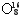B.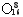C.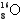D.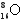13. An isotope of lithium contains 3 protons and 4 neutrons.  What is the correct notation for this lithium isotope?

A.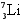B.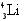C.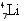D.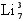14.Two atoms that are isotopes of an element contain _____.

A.  the same number of electrons and neutrons.

B.  the same number of protons and neutrons.

C.  the same number of protons and a different number of neutrons.

D.  different numbers of protons.

15.Which of the following is an isotope of iodine-13116.An isotope of sodium contains 11 protons and 12 neutrons.

A.  The atom also contains 11 electrons.

B.  The atom also contains 12 electrons.

C.  The atom also contains 23 electrons.

D.  There are no other factors related to the 11 protons and 12 neutrons.

17. Atoms of elements belonging to the same group have an identical number of

A. total electrons.

B. energy levels.

C. inner electrons.

D. valence electrons.

18.Which group contains the alkali metals?

A.  1A

B.  2A

C.  3A

D.  8A

19.Which statement is correct?

A.  Cr is in period 6B.

B.  Fe is a transition metal.

C.  Mg is an alkali metal.

D.  Cl is an inert gas

20.Arrange the following elements in order of increasing atomic radius: K, Mg, B.

A. K<Mg<B

B. B<Mg<K

C. Mg<K<B

D. B<K<Mg

## Solution 5 (1 Ratings )

Solved
Chemistry 1 Year Ago 41 Views